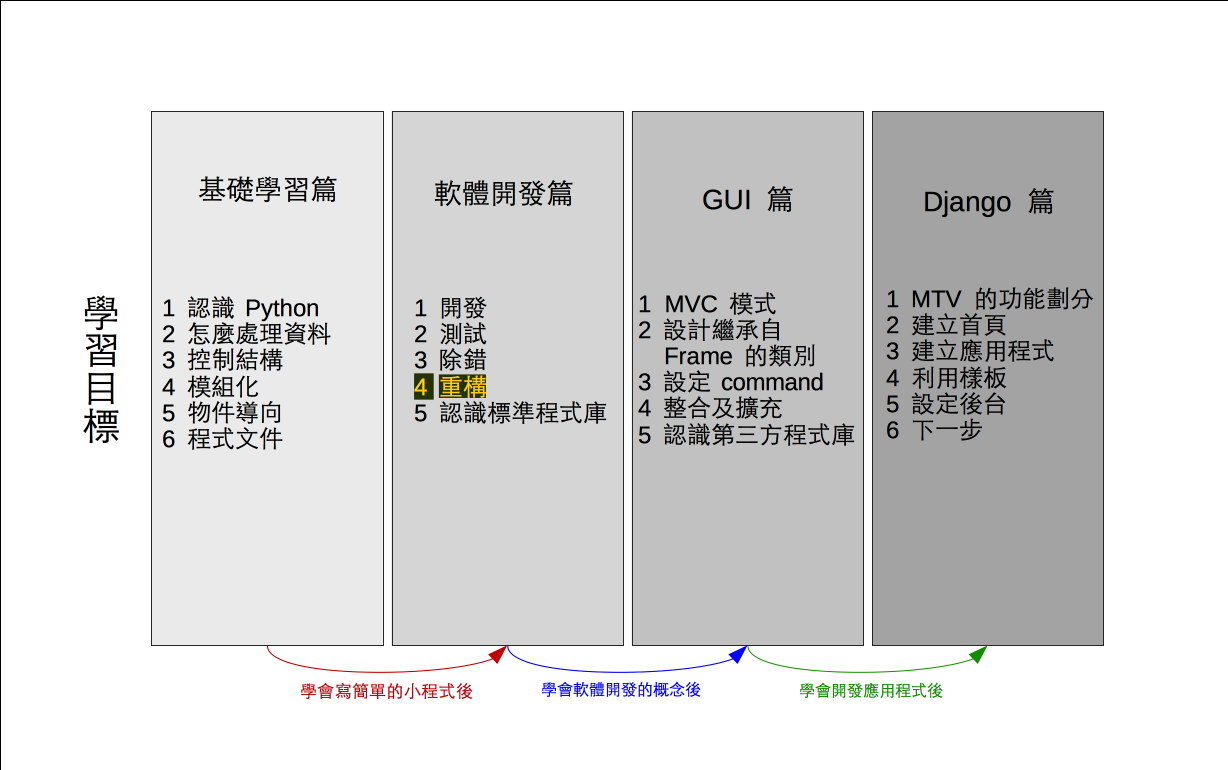# 單元 20 - 重構

～～學習進度表～～Source code → Refactoring
Maintainability
Extensibility

• 屬性 (attribute) 進行封裝 (encapsulation) ，並將屬性及方法 (method) 名稱更改為具有一致性。
• 移動屬性或方法，讓其出現在更適合的位置。
• 使型態 (type) 可以更具通用性，或挑出共通的屬性、方法成父類別 (superclass) 及子類別 (subclass) 。

``````def __init__(self):
self.setcode()``````

``````# 建立 Encrypt 物件同時建立密碼表
def __init__(self, str=None):
# 設定 code
if str == None:
self.code = [chr(i) for i in
range(97, 123)]
shuffle(self.code)
else:
self.code = list(str)

# 設定 alph
self.alph = [chr(i) for i in
range(97, 123)]``````

code 屬性改成串列的原因很簡單，因為串列是 Python 的工作馬，幾乎程式裡大大小小所有的工作都交給串列比較方面，另外串列是可變的，這給了我們很大的方便，留意這兩行

``````self.code = [chr(i) for i in
range(97, 123)]``````

``shuffle(self.code)``

shuffle() 直接攪亂串列裡元素的順序，同樣在 random 模組 (module) 中，所以要先 import 進來

``from random import shuffle``

``````# 回傳密碼表字串
def __str__(self):
code = "".join(self.code)
return "code: " + code``````

toEncode() 重構版本的編碼迴圈 (loop) 如下

``````# 利用迴圈走完參數字串的所有字元
for i in str:
# 判斷該字元是否為英文小寫字母
# 若是英文小寫字母就進行編碼轉換
if i in self.code:
j = self.alph.index(i)
result += self.code[j]
else:
result += i``````

``````# 利用迴圈走完參數字串的所有字元
for i in str:
# 判斷該字元是否為英文小寫字母
# 若是英文小寫字母就進行解碼轉換
if i in self.code:
j = self.code.index(i)
result += self.alph[j]
else:
result += i``````

1. 重構是指重新整理程式碼，讓程式更易於維護。
2. 常見的重構技術包括整理屬性、方法，挑出類別的共通特性定義父類別等等。
3. Encrypt 類別的重構包括刪去 setcode() ，重新定義 __init__() ，增加 __str__() ，修改 toEncode()toDecode() 等。

1. 什麼是重構？為什麼要替開發好的程式進行重構？
2. Encrppt 類別進行了哪些重構？為什麼要作這些重構？

1. 承接上一個單元的猜數字遊戲，將新程式寫在 exercise2001.py 中，替 __init__() 新增一個實體屬性 length ，並用參數 digit 來設定， digit 預設為 None ，當 digitNone 或小於 3 、大於 6 之時， length 就設定為 4 ，不然就設定為 digit
2. 承上題，將新程式寫在 exercise2002.py 中，修正 set_code() 裡的一個潛在錯誤，讓 GuessGame 能符合用 digit 設定猜測長度的設定。